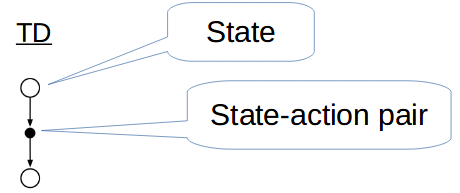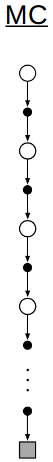# Sutton - TD Learning - Ch. 6

Posted on December 1, 2018 at 20:50

TL;DR -

#### Key Points

• In TD, as opposed to MC, “each error is proportional to the change over time of the prediction, that is, to the temporal differences in predictions.”
• TD is faster than MC, complete, and requires less variance (better batch training performance.

#### Questions

• TD Prediction update: $V(S_t) + \alpha[R_{t+1} + \gamma V(S_{t+1}) - V(S_t)]$ where the TD error $\delta_t = R_{t+1} + \gamma V(S_{t+1}) - V(S_t)$. So basically instead of knowing a model of the whole env. with prob. dist., we can estimate what our next reward will be.
• TD methods update estimate based on other estimate -> guess from a guess –> Bootsrtaping.
• $TD(0)$ guaranteed
• Backup Diagrams: Notice how the MC is so long because it has to know end state.• MRP: Markov decision process w/o actions.
• Under batch training, TD also performs better than MC.
# Batch training pseudocode
D = get(experience_dataset)
V = np.random() # Init. V arb.
# Repeat until converged
while delta > eps:
V_p = V
for (state, action, next_state, reward) in D:
V_p[state] = V_p[state] + alpha * (reward + (gamma * V_p[next_state]) - V[state])
V = V_p

• “The maximum-likelihood estimate of a parameter is the parameter value whose probability of generating the data is greatest.” For TD, associated expected reward is the average of the rewards observed.
• SARSA: On-Policy TD Control which converges for any $\epsilon$-soft policy ($\epsilon > 0$).

#### Exercises

• 3.Agent decided to go left and got 0, from update f-n ($0.9V(A) = 0.9*0.5$) –> 0.45
• 4.Not really, MC only good to a point and may not be able to reach same values of TD since it needs to explore world model first, so more walks/steps/Episodes always needed.
• 5.TD becomes more sensitive to changes due to higher alpha, so error may be more ‘drastic’ if a bad action taken.
• 6.Expected value.